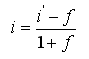HOMER Pro 3.13

 Navigation: Glossary Real Discount RateType: Input Variable Units: % Symbol: i

The real discount rate is used to convert between one-time costs and annualized costs. HOMER calculates the annual real discount rate (also called the real interest rate or interest rate) from the "Nominal discount rate" and "Expected inflation rate" inputs. HOMER uses the real discount rate to calculate discount factors and annualized costs from net present costs.

You can enter the nominal discount rate and the expected inflation rate in the Economics page under the Projects tab. HOMER uses the following equation to calculate the real discount rate:where: i = real discount rate i' = nominal discount rate (the rate at which you could borrow money) f = expected inflation rate

For example, if the nominal discount rate is 8% and the expected inflation rate is 3.5%, the annual real discount rate is 4.35%. If you want to enter the real annual interest rate directly (for example, to perform a sensitivity analysis), you can set the expected inflation rate to zero and enter values for the real discount rate into the nominal discount rate input.

By defining the real discount rate in this way, inflation is factored out of the economic analysis. All costs, therefore, become real costs, which are in defined in terms of constant dollars. The assumption is that the rate of inflation is the same for all costs.